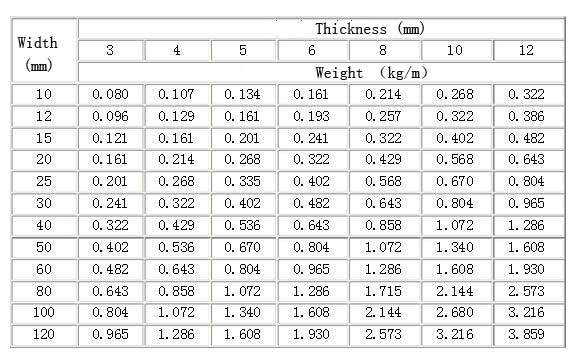# What is the weight per meter of aluminum strip

aluminium strip weight per meter

The weight of aluminum strip is easily calculated. Simply multiply the appropriate alloy density (see chart below) by the length, width, and thickness of the required part (see worked example below and note that we have used the density of alloy 6061 as the basis for our calculations).
For imperial weight calculations certain measurements (fractions) need to be converted to decimal inches.For an accurate calculation it is also important to allow for the rolling tolerance which affects the thickness and the cutting tolerances which affect the width and length. These vary from thickness to thickness – please contact us for details

aluminium strip weight per meter